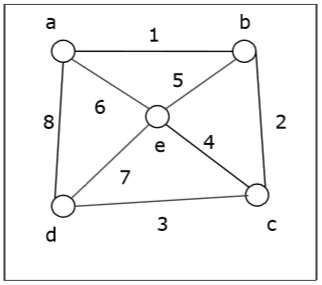Graph traversal is the problem of visiting all the vertices of a graph in some systematic order. There are mainly two ways to traverse a graph.

• Depth First Search

Breadth First Search (BFS) starts at starting level-0 vertex X of the graph G. Then we visit all the vertices that are the neighbors of X. After visiting, we mark the vertices as "visited," and place them into level-1. Then we start from the level-1 vertices and apply the same method on every level-1 vertex and so on. The BFS traversal terminates when every vertex of the graph has been visited.

BFS Algorithm

The concept is to visit all the neighbor vertices before visiting other neighbor vertices of neighbor vertices.

• Initialize status of all nodes as “Ready”.

• Put source vertex in a queue and change its status to “Waiting”.

• Repeat the following two steps until queue is empty −

• Remove the first vertex from the queue and mark it as “Visited”.

• Add to the rear of queue all neighbors of the removed vertex whose status is “Ready”. Mark their status as “Waiting”.

Problem

Let us take a graph (Source vertex is ‘a’) and apply the BFS algorithm to find out the traversal order.Solution

• Initialize status of all vertices to “Ready”.

• Put a in queue and change its status to “Waiting”.

• Remove a from queue, mark it as “Visited”.

• Add a’s neighbors in “Ready” state b, d and e to end of queue and mark them as “Waiting”.

• Remove b from queue, mark it as “Visited”, put its “Ready” neighbor c at end of queue and mark c as “Waiting”.

• Remove d from queue and mark it as “Visited”. It has no neighbor in “Ready” state.

• Remove e from queue and mark it as “Visited”. It has no neighbor in “Ready” state.

• Remove c from queue and mark it as “Visited”. It has no neighbor in “Ready” state.

• Queue is empty so stop.

So the traversal order is −

a → b → d → e → c

The alternate orders of traversal are −

a → b → e → d → c

Or, a → d → b → e → c

Or, a → e → b → d → c

Or, a → b → e → d → c

Or, a → d → e → b → c

Application of BFS

• Finding the shortest path
• Minimum spanning tree for un-weighted graph